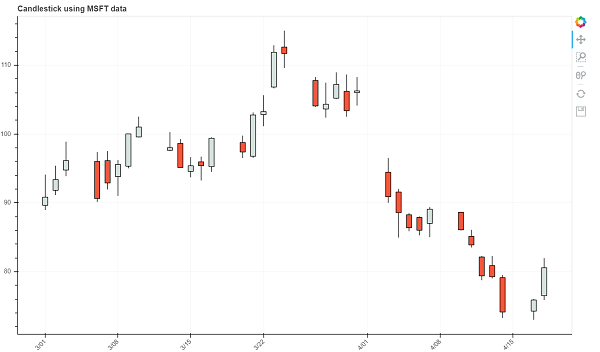# How can Bokeh be used to generate candle stick plot in Python?

PythonServer Side ProgrammingProgramming

#### Beyond Basic Programming - Intermediate Python

Most Popular

36 Lectures 3 hours

#### Practical Machine Learning using Python

Best Seller

91 Lectures 23.5 hours

#### Practical Data Science using Python

22 Lectures 6 hours

Bokeh is a Python package that helps in data visualization. It is an open source project. Bokeh renders its plot using HTML and JavaScript. This indicates that it is useful while working with web−based dashboards.

Bokeh can be easily used in conjunction with NumPy, Pandas, and other Python packages. It can be used to produce interactive plots, dashboards, and so on.

Dependencies of Bokeh −

Numpy
Pillow
Jinja2
Packaging
Pyyaml
Six
Python−dateutil

Installation of Bokeh on Windows command prompt

pip3 install bokeh

Installation of Bokeh on Anaconda prompt

conda install bokeh

Following is an example −

## Example

from math import pi
import pandas as pd
from bokeh.plotting import figure, output_file, show
from bokeh.sampledata.stocks import MSFT
my_df = pd.DataFrame(MSFT)[:35]
my_df["date"] = pd.to_datetime(my_df["date"])

inc = my_df.close > my_df.open
dec = my_df.open > my_df.close
w = 12*60*60*1000
TOOLS = "pan,wheel_zoom,box_zoom,reset,save"
p = figure(x_axis_type="datetime", tools=TOOLS, plot_width=1000, title = "Candlestick using MSFT data")
p.xaxis.major_label_orientation = pi/4
p.grid.grid_line_alpha=0.3

p.segment(my_df.date, my_df.high, my_df.date, my_df.low, color="black")
p.vbar(my_df.date[inc], w, my_df.open[inc], my_df.close[inc], fill_color="#D5E1DD", line_color="black")
p.vbar(my_df.date[dec], w, my_df.open[dec], my_df.close[dec], fill_color="#F2583E", line_color="black")

output_file("candlestick.html", title="candlestick plot")

show(p)

Note − To run this code, the pre−requisites are to install Bokeh, and execute the below command to download the sample datasets.

bokeh.sampledata.download()

## Output## Explanation

• The required packages are imported, and aliased.

• The MSFT data is an inbuilt dataset present in Bokeh library.

• It is stored as a dataframe.

• The figure function is called along with plot width and height.

• The ‘output_file’ function is called to mention the name of the html file that will be generated.

• The ‘TOOLS’ attribute is defined.

• The ‘vbar’ function present in Bokeh is called, along with data.

• The ‘show’ function is used to display the plot.